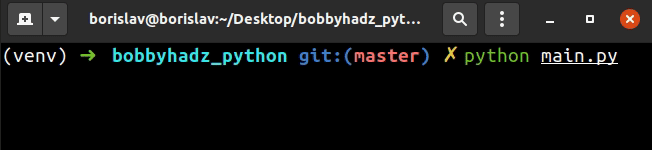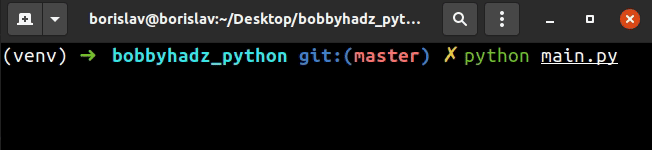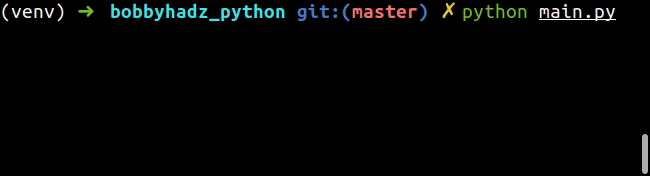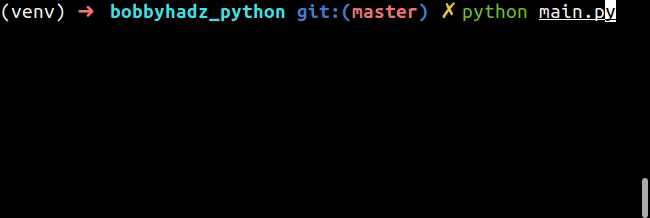# How to take Integer user input in PythonLast updated: Feb 20, 2023
3 min## #Take integer user input in Python

To take an integer user input:

1. Use the `input()` function to take input from the user.
2. Use a `try/except` statement to make sure the input value is an integer.
3. Use the `int()` class to convert the string to an integer.
main.py
```Copied!```# ✅ Take user input integer value
user_input = int(input('Enter an integer: '))
print(user_input)

# ------------------------------------------------

# ✅ Take user input integer value with validation
try:
user_input = int(input('Enter an integer: '))
print(user_input)
except ValueError:
print('Enter a valid integer')
``````We used the `input()` function to take user input.

The input function takes an optional `prompt` argument and writes it to standard output without a trailing newline.

The function then reads the line from the input, converts it to a string and returns the result.

Note that the `input` function is guaranteed to return a string, even if the user entered an integer.

This is why we used the int() class to convert the value to an integer.

main.py
```Copied!```user_input = int(input('Enter an integer: '))
print(user_input)
``````

If the user enters an invalid integer, we'd get a `ValueError`.

You can handle the error using a try/except statement.

main.py
```Copied!```try:
user_input = int(input('Enter an integer: '))
print(user_input)
except ValueError:
print('Enter a valid integer')
``````If the user enters an invalid integer, the `int()` class throws a `ValueError` which gets handled in the `except` block.

## #Only allow Integer user input in Python

To only allow integer user input:

1. Use a `while True` loop to loop until the user enters an integer.
2. Use the `int()` class to attempt to convert the value the user entered to an integer.
3. If the user entered an integer, use the `break` statement to break out of the loop.
main.py
```Copied!```while True:
try:
num = int(input('Your favorite integer: '))
print(num)
break
except ValueError:
``````We used a `while True` loop to iterate until the user enters an integer value.

The ` input()` function takes an optional `prompt` argument and writes it to standard output without a trailing newline.

The function then reads the line from the input, converts it to a string and returns the result.

The `input()` function always returns a string, even if the user enters an integer.

We used the `int()` class to try to convert the value the user entered to an integer.

main.py
```Copied!```while True:
try:
num = int(input('Your favorite integer: '))
print(num)
break
except ValueError:
``````

If the attempt to convert the value fails, a `ValueError` is raised and the `except` block runs.

The `except` block prints the "Please enter an integer" message and the loop re-runs.

If the user enters an integer, we print the integer and `break` out of the `while` loop.

The break statement breaks out of the innermost enclosing `for` or `while` loop.

## #Only allow integer user input in a given range

If you need to make sure the user enters an integer in a given range, use an `if` statement.

main.py
```Copied!```while True:
try:
num = int(input('Integer between 1 and 100: '))
print(num)

if num < 1 or num > 100:
raise ValueError

break
except ValueError:
print('Please enter an integer between 1 and 100.')
``````The code snippet only allows a user to enter an integer between `1` and `100`.

If the value is not an integer, is less than `1` or is greater than `100`, a `ValueError` is raised and the loop re-runs.

Once the user enters an integer in the specified range, no `ValueError` is raised and we break out of the `while` loop.